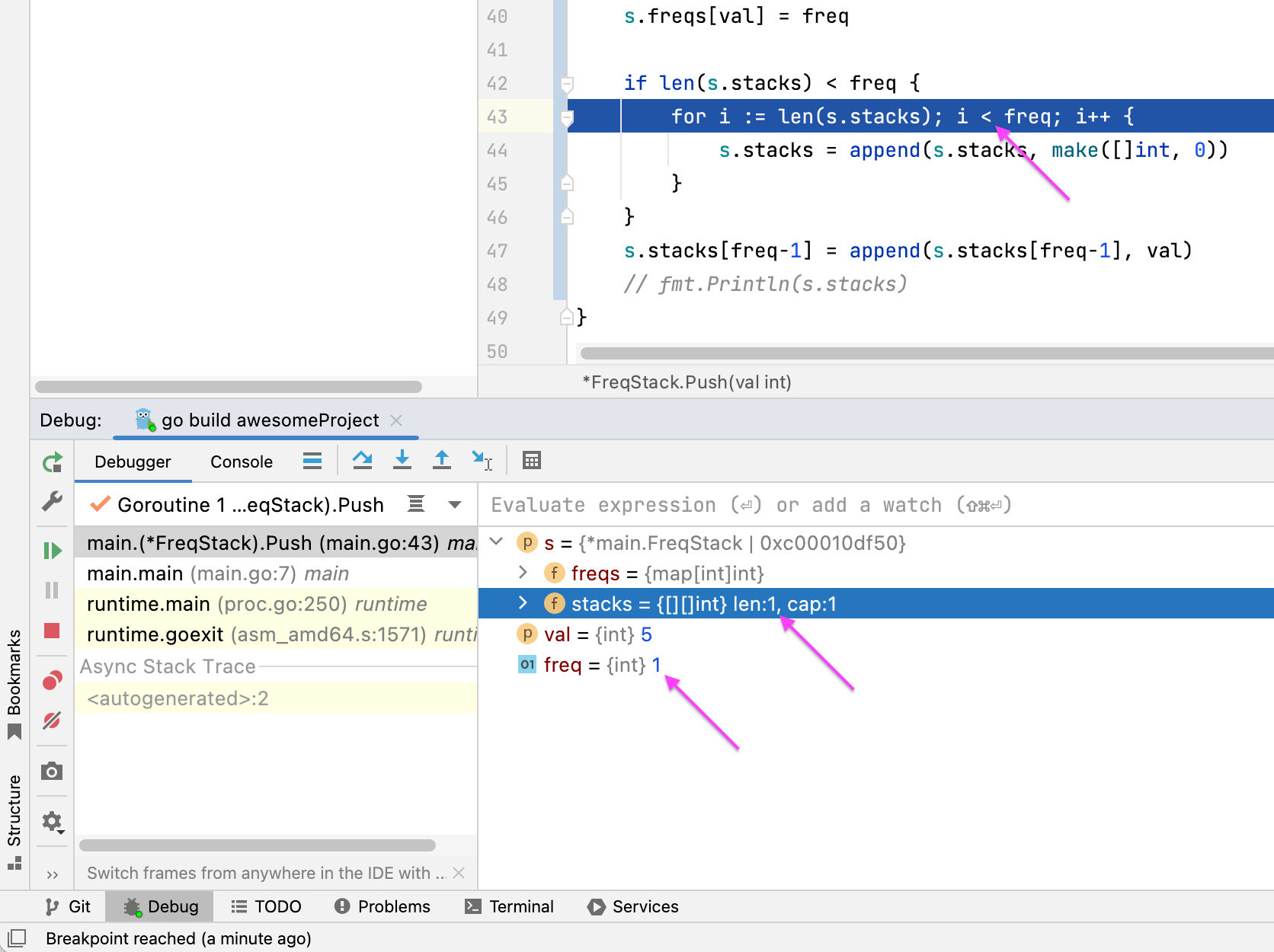https://vimeo.com/717251704

`package mainimport "fmt"func main() {   freqStack := Constructor()   freqStack.Push(5) // The stack is    freqStack.Push(7) // The stack is [5,7]   freqStack.Push(5) // The stack is [5,7,5]   freqStack.Push(7) // The stack is [5,7,5,7]   freqStack.Push(4) // The stack is [5,7,5,7,4]   freqStack.Push(5) // The stack is [5,7,5,7,4,5]   var x int   x = freqStack.Pop() // return 5, as 5 is the most frequent. The stack becomes [5,7,5,7,4].   fmt.Println(x)   x = freqStack.Pop() // return 7, as 5 and 7 is the most frequent, but 7 is closest to the top. The stack becomes [5,7,5,4].   fmt.Println(x)   x = freqStack.Pop() // return 5, as 5 is the most frequent. The stack becomes [5,7,4].   fmt.Println(x)   x = freqStack.Pop() // return 4, as 4, 5 and 7 is the most frequent, but 4 is closest to the top. The stack becomes [5,7].   fmt.Println(x)}type FreqStack struct {   freqs  map[int]int   stacks [][]int}func Constructor() FreqStack {   return FreqStack{      freqs:  make(map[int]int),      stacks: make([][]int, 0),   }}func (s *FreqStack) Push(val int) {   freq := s.freqs[val]   freq++   s.freqs[val] = freq   if len(s.stacks) < freq {      for i := len(s.stacks); i < freq; i++ {         s.stacks = append(s.stacks, make([]int, 0))      }   }   s.stacks[freq-1] = append(s.stacks[freq-1], val)   // fmt.Println(s.stacks)}func (s *FreqStack) Pop() int {   n := len(s.stacks)   lastStack := s.stacks[n-1]   value := lastStack[len(lastStack)-1]   s.freqs[value]--   s.stacks[n-1] = s.stacks[n-1][:len(lastStack)-1]   if len(s.stacks[n-1]) == 0 {      s.stacks = s.stacks[:n-1]   }   return value}`
4 条评论On the second iteration, as you can see, len(s.stacks) is equal to 1 and freq is equal to 1 too, so condition i < freq returns false.GoLand behaves as expected in that case and returns from the loop as well as from the if condition.Thanks for your reply, sorry there might be some confusion in the video. but I'm not talking about the jumps in iterations.

My question is why is it jumping from line 40 to line 37.In fact, there are two confusing jumps (see video).

38 --> 37  --> 38 --> 39 --> 40 --> 37Thanks for the details.

We've filled a new ticket on Delve tracker: https://github.com/go-delve/delve/issues/3033